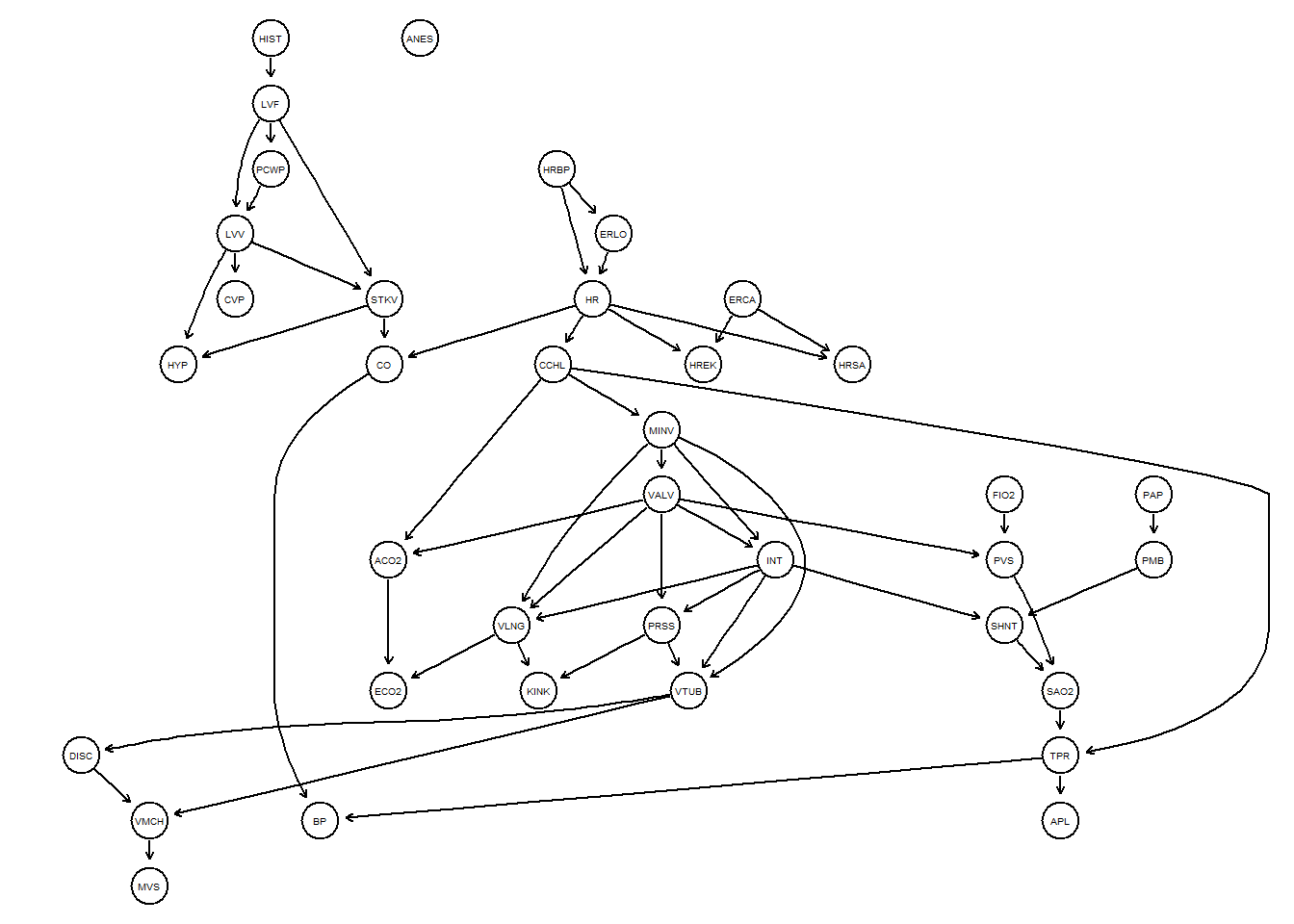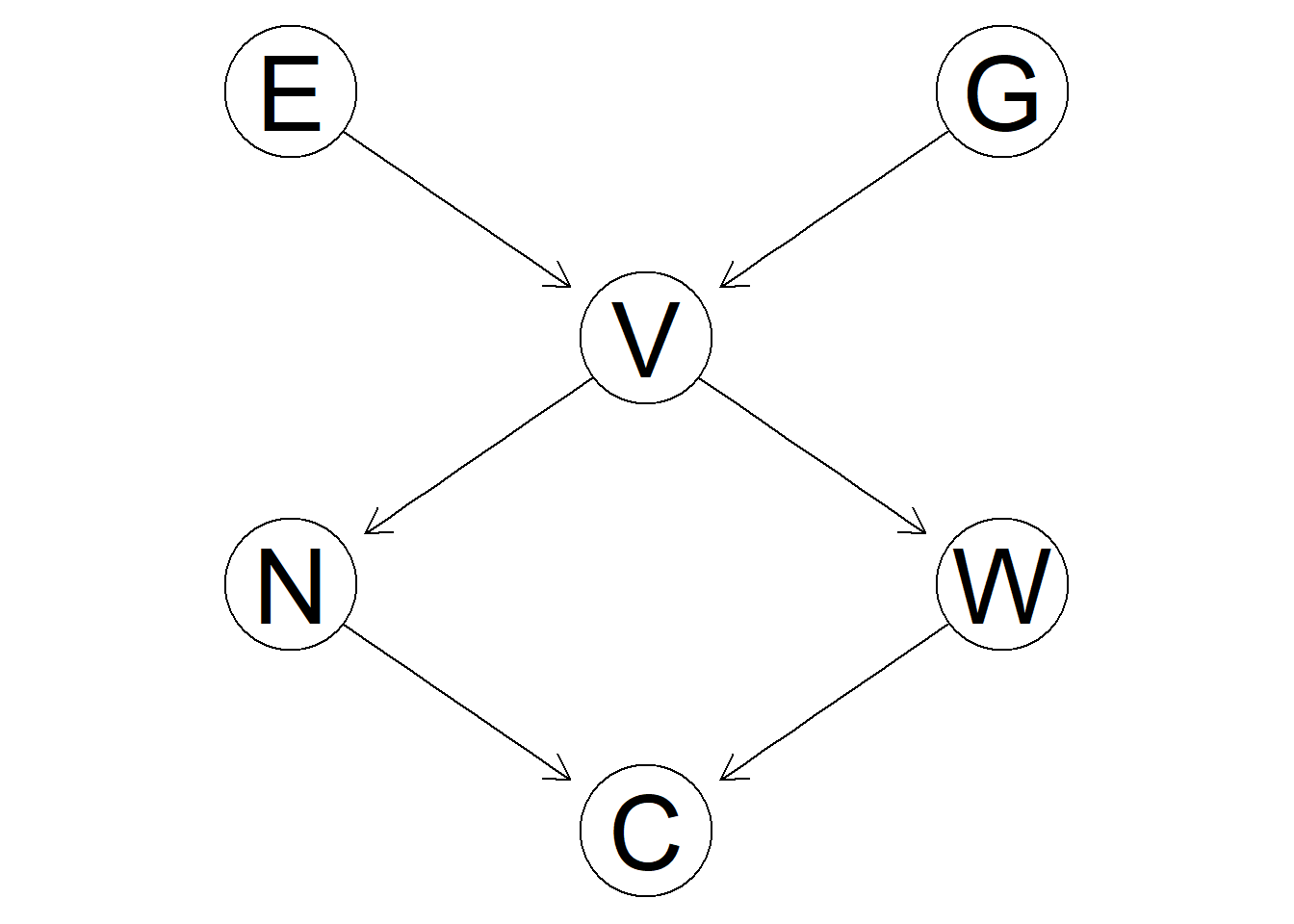# 贝叶斯网络-学习笔记bnlearn

bnlearn
Published

January 17, 2023

``library(bnlearn)``
``Warning: package 'bnlearn' was built under R version 4.2.2``
``````dag<-empty.graph(nodes=c('A','S','E','O','R','T'))
arcs.set<-matrix(c("A", "E", "S", "E", "E", "O", "E", "R", "O", "T", "R", "T"), byrow = TRUE, ncol = 2, dimnames = list(NULL, c("from", "to")))
arcs(dag)<-arcs.set
dag``````
``````
Random/Generated Bayesian network

model:
[A][S][E|A:S][O|E][R|E][T|O:R]
nodes:                                 6
arcs:                                  6
undirected arcs:                     0
directed arcs:                       6
average markov blanket size:           2.67
average neighbourhood size:            2.00
average branching factor:              1.00

generation algorithm:                  Empty ``````
``arcs(dag)``
``````     from to
[1,] "A"  "E"
[2,] "S"  "E"
[3,] "E"  "O"
[4,] "E"  "R"
[5,] "O"  "T"
[6,] "R"  "T"``````
``modelstring(dag)``
`` "[A][S][E|A:S][O|E][R|E][T|O:R]"``
``````learned<-hc(alarm)
learned``````
``````
Bayesian network learned via Score-based methods

model:
[HIST][HRBP][PAP][FIO2][ANES][ERCA][LVF|HIST][PMB|PAP][ERLO|HRBP][PCWP|LVF]
[HR|HRBP:ERLO][HREK|HR:ERCA][HRSA|HR:ERCA][LVV|PCWP:LVF][CCHL|HR][CVP|LVV]
[MINV|CCHL][STKV|LVF:LVV][CO|STKV:HR][HYP|LVV:STKV][VALV|MINV][INT|MINV:VALV]
[VMCH|DISC:VTUB][MVS|VMCH]
nodes:                                 37
arcs:                                  53
undirected arcs:                     0
directed arcs:                       53
average markov blanket size:           3.46
average neighbourhood size:            2.86
average branching factor:              1.43

learning algorithm:                    Hill-Climbing
score:                                 BIC (disc.)
penalization coefficient:              4.951744
tests used in the learning procedure:  2718
optimized:                             TRUE ``````
``graphviz.plot(learned)``
``Loading required namespace: Rgraphviz````````dag.bnlearn <- model2network("[G][E][V|G:E][N|V][W|V][C|N:W]")
dsep(dag.bnlearn,'G','V')``````
`` FALSE``
``graphviz.plot(dag.bnlearn)````dsep(dag.bnlearn, "E", "V", "V")``
`` TRUE``